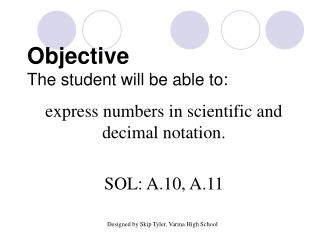DownloadDownload PresentationObjective The student will be able to:

# Objective The student will be able to:

Télécharger la présentation## Objective The student will be able to:

- - - - - - - - - - - - - - - - - - - - - - - - - - - E N D - - - - - - - - - - - - - - - - - - - - - - - - - - -
##### Presentation Transcript

1. ObjectiveThe student will be able to: express numbers in scientific and decimal notation. SOL: A.10, A.11 Designed by Skip Tyler, Varina High School

2. AIM: What is scientific notation and how do I apply it? • Do Now: Take out last night’s assignment, please. If you need an extra copy, please raise your hand.

3. HW Review: • Regents Review 7 is due the Wednesday that we return from break • You will receive a Spring Break packet on Thursday

4. Big Ideas: • Scientific Notation helps us express really big numbers in a more manageable way • You can use scientific notation everywhere • Have you used it somewhere before?

5. Notes: Scientific Notation A number is expressed in scientific notation when it is in the form a x 10n where a is between 1 and 10 and n is an integer

6. Part I: Writing numbers in Scientific Notation: How wide is our universe? 210,000,000,000,000,000,000,000 miles (22 zeros) This number is written in decimal notation. When numbers get this large, it is easier to write them in scientific notation.

7. 2.10,000,000,000,000,000,000,000. How many decimal places did you move the decimal? 23 When the original number is more than 1, the exponent is positive. The answer in scientific notation is 2.1 x 1023

8. 1) Express 0.0000000902 in scientific notation. Where would the decimal go to make the number be between 1 and 10? 9.02 The decimal was moved how many places? 8 When the original number is less than 1, the exponent is negative. 9.02 x 10-8

9. Write 28750.9 in scientific notation. • 2.87509 x 10-5 • 2.87509 x 10-4 • 2.87509 x 104 • 2.87509 x 105

10. Part 2: Writing in Decimal Notation 2) Express 1.8 x 10-4 in decimal notation. 0.00018 3) Express 4.58 x 106 in decimal notation. 4,580,000

11. Classwork: • Now, we’re going to examine how we apply scientific notation in our daily lives.

12. Our solar system:

13. Your task: • First, rewrite the distance between the sun and the planets in scientific notations • Then, you’re going to compare them to distances between NYC and other cities around the world • Then, you need to decide the closest and furthest cities from NYC which are in scientific notation already.

14. During group work time: • Work as quickly and quietly as possible • If you have a question, first, ask a groupmate, then, if your group still does not know, ask me by raising your hand. • You will return this assignment to me by the end of class for a grade.

15. Summary: • Why is scientific notation important? • How can you apply scientific notation in your daily life?

16. 4) Use a calculator to evaluate: 4.5 x 10-5 1.6 x 10-2 Type 4.5 -5 1.6 -2 You must include parentheses if you don’t use those buttons!! (4.5 x 10 -5) (1.6 x 10 -2) 0.0028125 Write in scientific notation. 2.8125 x 10-3

17. 5) Use a calculator to evaluate: 7.2 x 10-9 1.2 x 102On the calculator, the answer is: 6.E -11 The answer in scientific notation is 6 x 10 -11 The answer in decimal notation is 0.00000000006

18. 6) Use a calculator to evaluate (0.0042)(330,000).On the calculator, the answer is 1386. The answer in decimal notation is 1386 The answer in scientific notation is 1.386 x 103

19. 7) Use a calculator to evaluate (3,600,000,000)(23).On the calculator, the answer is: 8.28 E +10 The answer in scientific notation is 8.28 x 10 10 The answer in decimal notation is 82,800,000,000

20. Write (2.8 x 103)(5.1 x 10-7) in scientific notation. • 14.28 x 10-4 • 1.428 x 10-3 • 14.28 x 1010 • 1.428 x 1011

21. Write in PROPER scientific notation.(Notice the number is not between 1 and 10) 8) 234.6 x 109 2.346 x 1011 9) 0.0642 x 104 on calculator: 642 6.42 x 10 2

22. Write 531.42 x 105 in scientific notation. • .53142 x 102 • 5.3142 x 103 • 53.142 x 104 • 531.42 x 105 • 53.142 x 106 • 5.3142 x 107 • .53142 x 108